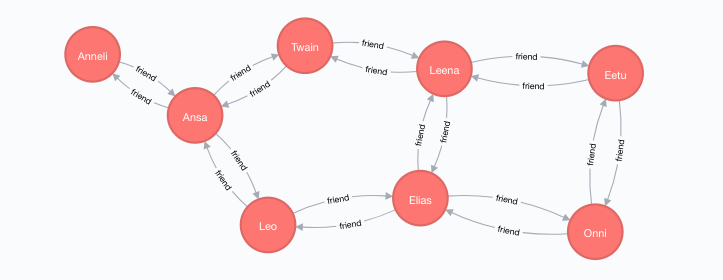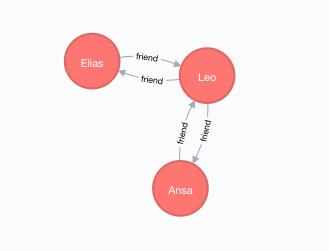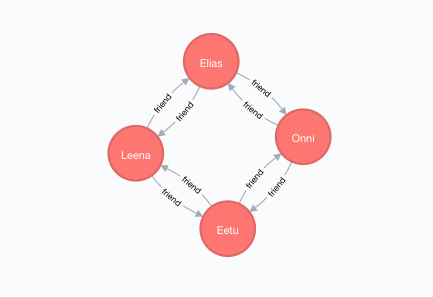# Finding Shortest Paths Between Two Nodes Of A Neo4j Graph Using Python

## Overview:

• In Graph Theory parlance, a node or vertex is the basic element of a Graph.
• The links that connect the nodes are called edges or relationships between the nodes.
• A graph will have a set of nodes and a set of relationships. A graph may not have any relationship at all in which case the set of relationships is an empty set.
• In a given Graph there could be several sequence of relationships leading from a source node to a destination node. The sequence which has the minimal sum of the weights assigned to its relationships among other sequences is the shortest path between the chosen nodes.
• A weight assigned to an edge provides some meaning to the edge. For example, the weight assigned to the edges of a graph could mean speed of a link between two communication end points, the density of the traffic on a road, width of a tunnel and so on.
• In case when equal weights are assigned to the relationships of the Graph it can be used to denote the number of hops, number of relays to be made and so on.
• The Cypher Query Language – CQL, has a built-in function called shortestPath() through which the shortest path can be found between nodes. The shortestPath()function takes a pattern which can comprise of the type of edge or relationship, number of hops in the path and the direction of the relationship.
• As of this writing the shortestPath()function assumes all the relationships of the graph is assigned equal weights. Also the CQL uses different algorithms based on the predicates used in the query resulting in different execution times.## Finding the Shortest Path between two nodes of a graph in Neo4j using CQL and Python:

• From a Python program import the GraphDatabase module, which is available through installing Neo4j Python driver.
• Create a database connection by creating a driver instance. The driver instance is capable of managing the connection pool requirements of the application.
• Create a session object and pass the CQL command to execute it on the database server.
• In this example below, two CQL commands are used.
• One is to find the shortest distance between two nodes
• Another is to find all the shortest distances between two nodes• To find the shortest distance the CQL function shortestPath() is used and to find all the shortest distances the CQL function  allShortestPaths() is used.## Example:

 # import the GraphDatabase module - the neo4j driver for Python from neo4j.v1 import GraphDatabase   # Provide Database Credentials uriToServer     = "bolt://localhost:7687" usr             = "neo4j" pwd             = "test"   # Obtain a driver instance connecting to neo4j database server graphDB_Driver  = GraphDatabase.driver(uriToServer, auth=(usr, pwd))   # Create a set of nodes representing friendship among a set of persons cqlCreateNodesAndEdegs    = """CREATE (Onni:person { name: "Onni"}),                                 (Elias:person { name: "Elias"}),                                 (Eetu:person { name: "Eetu"}),                                 (Leo:person { name: "Leo"}),                                 (Leena:person { name: "Leena"}),                                 (Twain:person { name: "Twain"}),                                 (Ansa:person { name: "Ansa"}),                                 (Anneli:person { name: "Anneli"}),                                 (Onni)-[:friend {miles: 259}]->(Eetu),                                 (Onni)-[:friend {miles: 259}]->(Elias),                                 (Elias)-[:friend {miles: 259}]->(Leo),                                 (Leena)-[:friend {miles: 259}]->(Elias),                                 (Eetu)-[:friend {miles: 259}]->(Leena),                                 (Leena)-[:friend {miles: 259}]->(Twain),                                 (Ansa)-[:friend {miles: 259}]->(Leo),                                 (Twain)-[:friend {miles: 259}]->(Ansa),                                 (Ansa)-[:friend {miles: 259}]->(Anneli),                                 (Onni)<-[:friend {miles: 259}]-(Eetu),                                 (Onni)<-[:friend {miles: 259}]-(Elias),                                 (Elias)<-[:friend {miles: 259}]-(Leo),                                 (Leena)<-[:friend {miles: 259}]-(Elias),                                 (Eetu)<-[:friend {miles: 259}]-(Leena),                                 (Leena)<-[:friend {miles: 259}]-(Twain),                                 (Ansa)<-[:friend {miles: 259}]-(Leo),                                 (Twain)<-[:friend {miles: 259}]-(Ansa),                                 (Ansa)<-[:friend {miles: 259}]-(Anneli)"""                     cqlShorestPath      = """MATCH (p1:person { name: 'Ansa' }),(p2:person { name: 'Elias' }), path = shortestPath((p1)-[*..15]-(p2))                       RETURN path"""                        cqlShorestPaths     = """MATCH (p1:person { name: 'Eetu' }),(p2:person { name: 'Elias' }), path = allShortestPaths((p1)-[*..15]->(p2))                       RETURN path"""   # Execute the CQL queries with graphDB_Driver.session() as graphDB_Session:     # Create nodes and edges     nodes = graphDB_Session.run(cqlCreateNodesAndEdegs)       # Find the shortest path between two nodes..in this case two people     shortestPath = graphDB_Session.run(cqlShorestPath)       print("Shortest path between nodes - Ansa and Elias:")     for record in shortestPath:         nodes = record["path"].nodes                 for node in nodes:             print(node)       # Find the shortest paths between two nodes     shortestPaths = graphDB_Session.run(cqlShorestPaths)       print("======")     print("Shortest paths between nodes - Eetu and Elias:")     pathCount = 0         for record in shortestPaths:         pathCount   = pathCount + 1         nodes       = record["path"].nodes           print("Path %d:"%(pathCount))         for node in nodes:             print(node)

## Output:

 Shortest path between nodes - Ansa and Elias: ====== Shortest paths between nodes - Eetu and Elias: Path 1: Path 2: